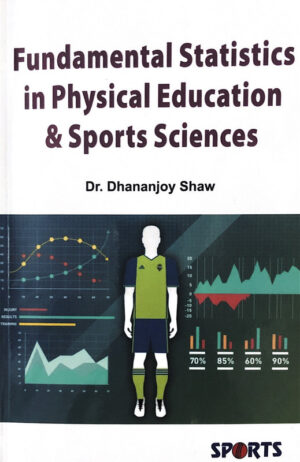Sale!

# Fundamental Statistics in Physical Education & Sports Sciences

About the book – Chapter I, deals with the introduction of statistics including the definition, history, need, importance, techniques and statistical process. – Chapter II, deals with Variables and attributes with their different types of classification and applicable concepts. – Chapter III, deals with the concept of frequency distribution and different methods of frequency table and distribution computations. – Chapter IV and V, deals with the measures of central tendency and variability, their concepts, types, applications and characteristics. Chapter VI, deals with the normal probability curve, the basic concept of probability, basic rules of probability, binomial distribution, characteristics and importance of normal distribution, divergence from normality, ‘t’ distribution for small samples, degree of freedom and confidence limits. – Chapter VII, discusses different type of scales, Z- scale, T- scale, 6- Sigma Scale and Hull Scale, Percentiles, Quartiles, Deciles, Percentile Rank, and finally concluded with a comparison among various scales. – Chapter VIII, deals with sampling, its reliability, theory and procedure. – Chapter IX, gives an introduction to bivariate and correlation statistics very briefly. – Chapter X, deals with testing of hypothesis or inferential stastistics reflecting on the differences between the statistics, null hypothesis, level of significance, errors of indifference, two-tail and one-tail tests, differences between means and power of statistical test. – Chapter XI, gives an introduction to non-parametric statistics emphasising on chi square, contingency, sign test, median test, tetracholicc correlation, eta-correlation and rho-correlation. – Chapter XII, deals with different types of graphs namely line graph, bar graph, pictograph, pie graph etc. – Chapter XIII, includes basic key concepts and practical applications of experimental design and concluded with concepts and misconnects of experimental design.?

360.00

SKU: Shaw-0570-H
Weight 300 kg 24 × 14 × 2 cm Dr. Dhananjoy Shaw Paperback First 2021 English Dr. Dhananjoy Shaw Sports Publication

## Reviews

There are no reviews yet.